# Artificial Neural Networks Artificial Neural Networks Interconnected networks

• Slides: 37Artificial Neural NetworksArtificial Neural Networks • Interconnected networks of simple units ("artificial neurons"). – Weight wij is the weight of the ith input into unit j. – Depending on the final output value we determine the class. – If more than one output units then we choose the one with the greatest value. • Learning takes place by adjusting the weights in the network – so that the desired output is produced whenever a training instance is presented.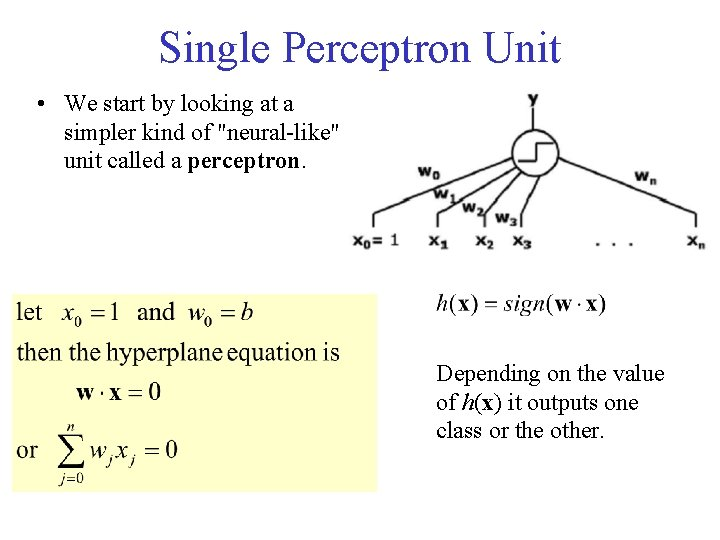Single Perceptron Unit • We start by looking at a simpler kind of "neural-like" unit called a perceptron. Depending on the value of h(x) it outputs one class or the other.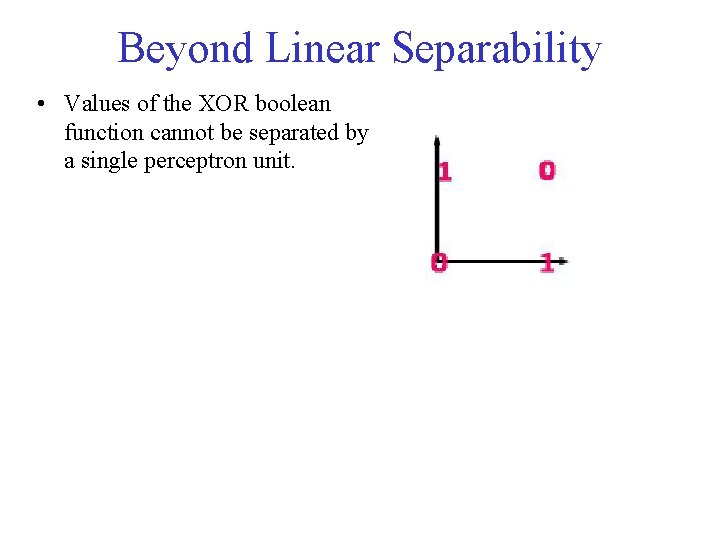Beyond Linear Separability • Values of the XOR boolean function cannot be separated by a single perceptron unit.Multi-Layer Perceptron • Solution: Combine multiple linear separators. • Introduction of "hidden" units into NN make them much more powerful: – they are no longer limited to linearly separable problems. • Earlier layers transform the problem into more tractable problems for the latter layers.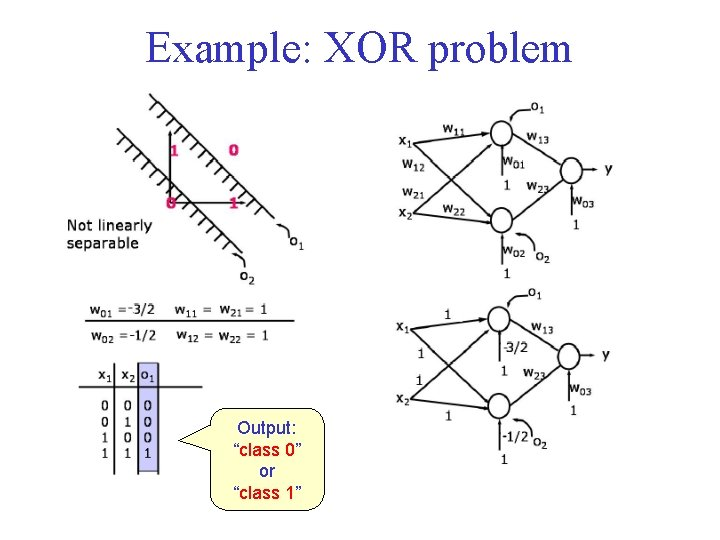Example: XOR problem Output: “class 0” or “class 1”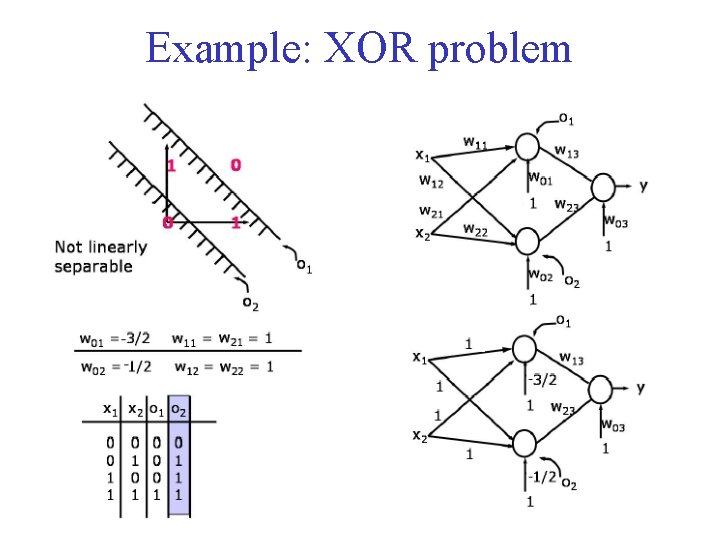Example: XOR problem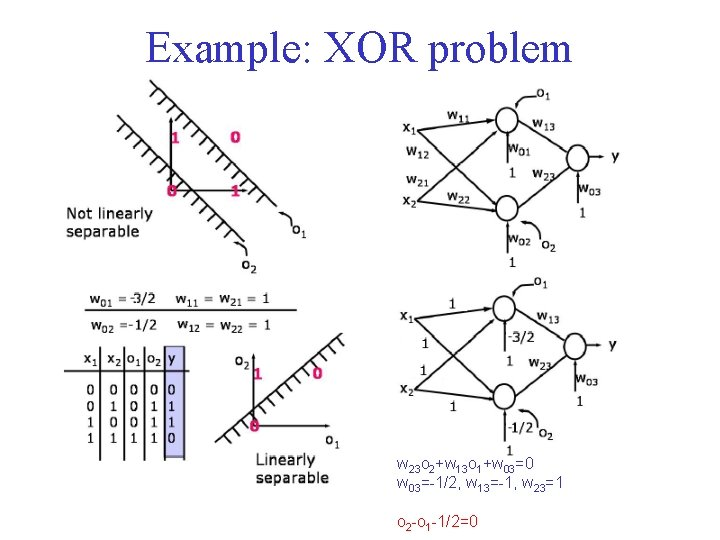Example: XOR problem w 23 o 2+w 13 o 1+w 03=0 w 03=-1/2, w 13=-1, w 23=1 o 2 -o 1 -1/2=0Multi-Layer Perceptron • Any set of training points can be separated by a three-layer perceptron network. • “Almost any” set of points is separable by a two-layer perceptron network.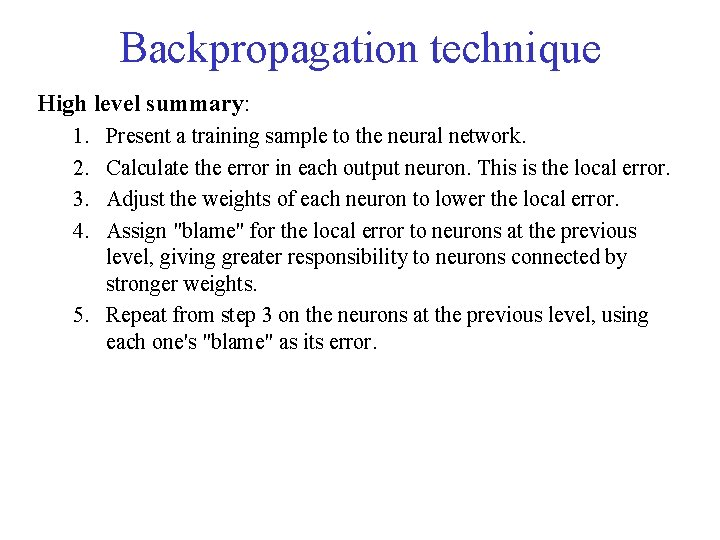Backpropagation technique High level summary: 1. 2. 3. 4. Present a training sample to the neural network. Calculate the error in each output neuron. This is the local error. Adjust the weights of each neuron to lower the local error. Assign "blame" for the local error to neurons at the previous level, giving greater responsibility to neurons connected by stronger weights. 5. Repeat from step 3 on the neurons at the previous level, using each one's "blame" as its error.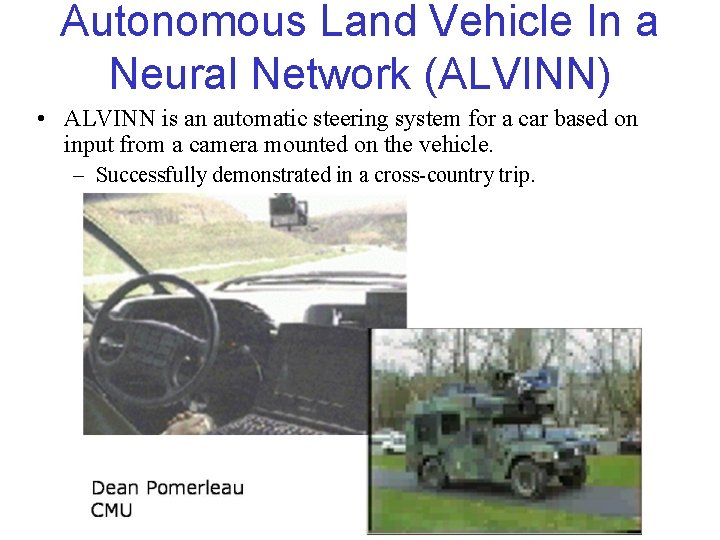Autonomous Land Vehicle In a Neural Network (ALVINN) • ALVINN is an automatic steering system for a car based on input from a camera mounted on the vehicle. – Successfully demonstrated in a cross-country trip.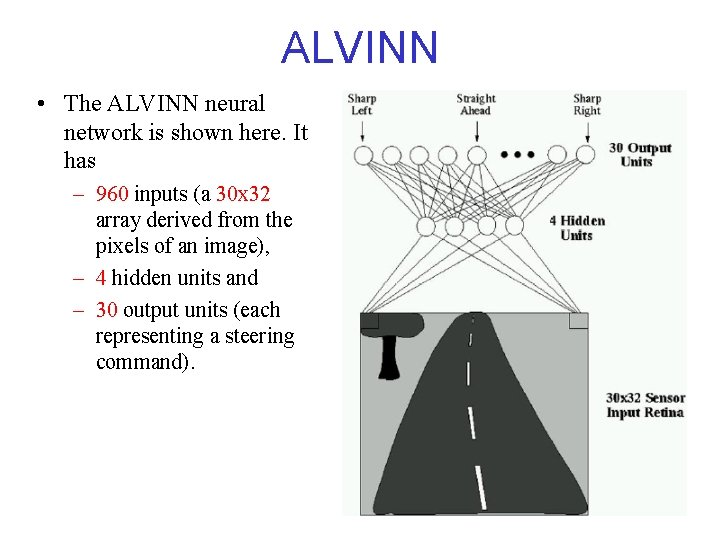ALVINN • The ALVINN neural network is shown here. It has – 960 inputs (a 30 x 32 array derived from the pixels of an image), – 4 hidden units and – 30 output units (each representing a steering command).SVMs vs. ANNs • Comparable in practice. • Some comment: "SVMs have been developed in the reverse order to the development of neural networks (NNs). SVMs evolved from the sound theory to the implementation and experiments, while the NNs followed more heuristic path, from applications and extensive experimentation to theory. “ (Wang 2005)Soft Threshold • A natural question to ask is whether we could use gradient ascent/descent to train a multi-layer perceptron. • The answer is that we can't as long as the output is discontinuous with respect to changes in the inputs and the weights. – In a perceptron unit it doesn't matter how far a point is from the decision boundary, we will still get a 0 or a 1. • We need a smooth output (as a function of changes in the network weights) if we're to do gradient descent.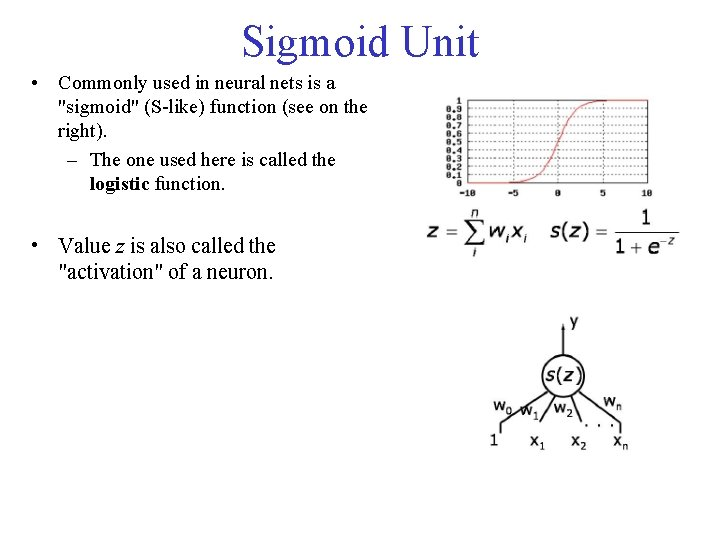Sigmoid Unit • Commonly used in neural nets is a "sigmoid" (S-like) function (see on the right). – The one used here is called the logistic function. • Value z is also called the "activation" of a neuron.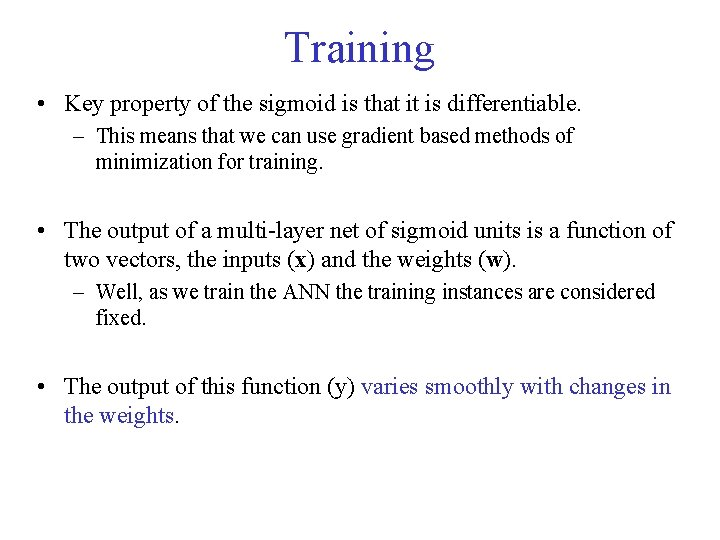Training • Key property of the sigmoid is that it is differentiable. – This means that we can use gradient based methods of minimization for training. • The output of a multi-layer net of sigmoid units is a function of two vectors, the inputs (x) and the weights (w). – Well, as we train the ANN the training instances are considered fixed. • The output of this function (y) varies smoothly with changes in the weights.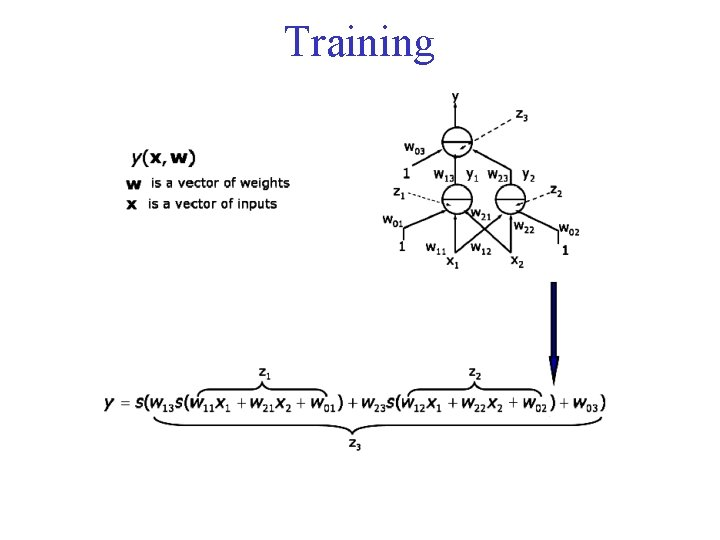Training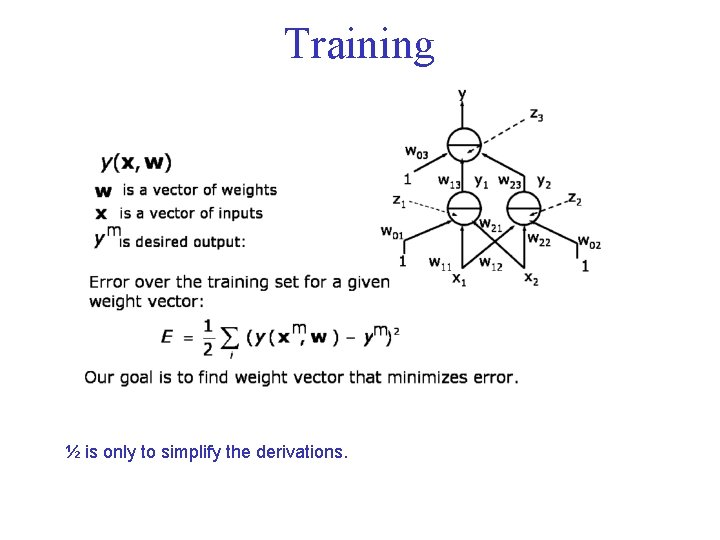Training ½ is only to simplify the derivations.Gradient Descent We follow gradient descent Online version: We consider each time only the error for one data item Gradient of the training error is computed as a function of the weights. As a shorthand, we will denote y(xm, w) just by y.Gradient Descent – Single Unit Substituting in the equation of previous slide we get (for the arbitrary ith element of w): Delta ruleDerivative of the sigmoid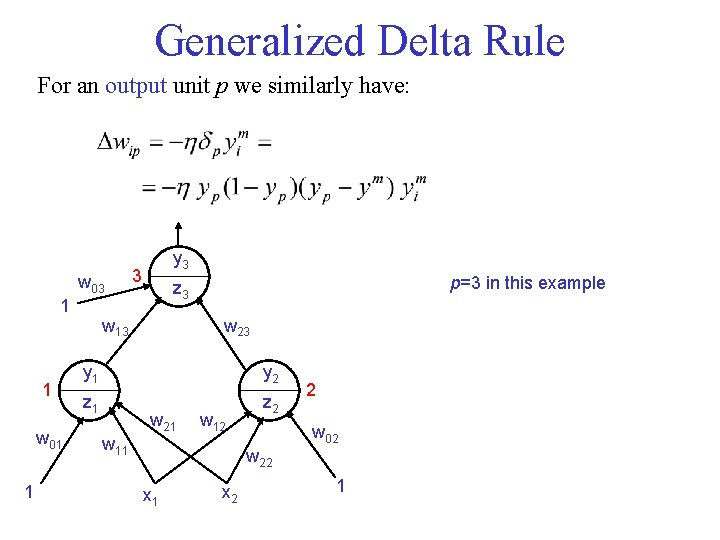Generalized Delta Rule For an output unit p we similarly have: w 03 1 1 w 01 1 y 3 3 p=3 in this example z 3 w 13 w 23 y 1 y 2 z 1 z 2 w 21 w 12 w 11 2 w 02 w 22 x 1 x 2 1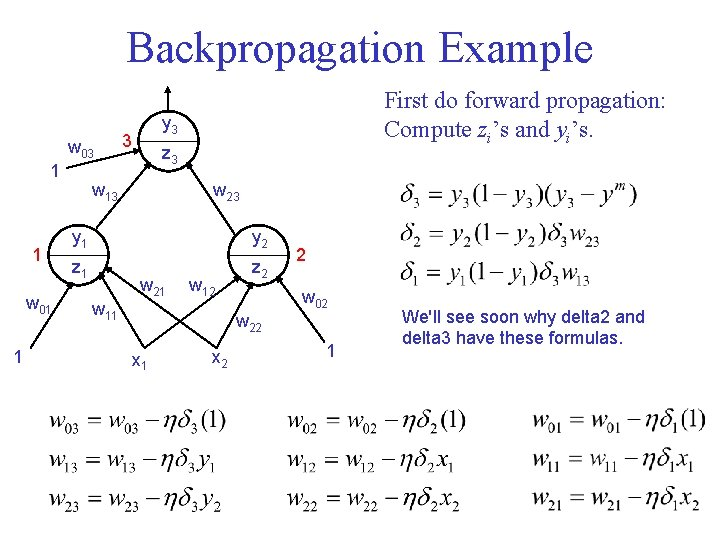Backpropagation Example w 03 1 1 w 01 1 First do forward propagation: Compute zi’s and yi’s. y 3 3 z 3 w 13 w 23 y 1 y 2 z 1 z 2 w 21 w 12 w 11 2 w 02 w 22 x 1 x 2 1 We'll see soon why delta 2 and delta 3 have these formulas.Deriving 2 and 2 3 w 03 1 1 w 01 1 y 3 z 3 w 13 w 23 y 1 y 2 z 1 z 2 w 21 w 12 w 11 2 w 02 w 22 x 1 x 2 We similarly derive delta 1. 1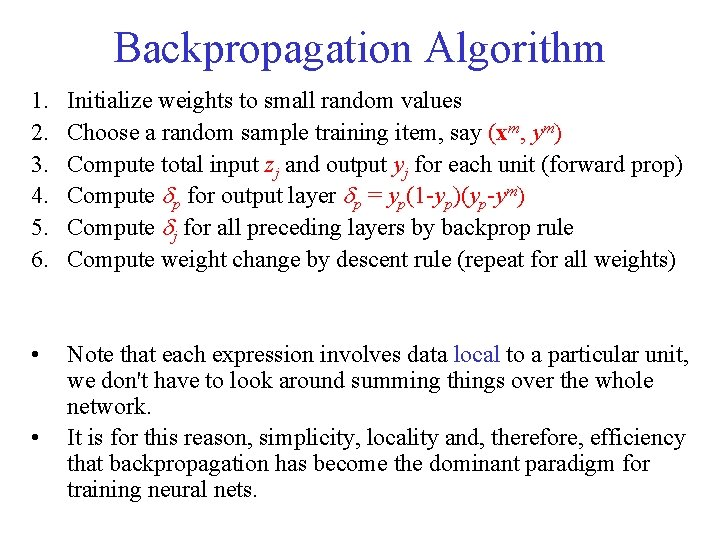Backpropagation Algorithm 1. 2. 3. 4. 5. 6. Initialize weights to small random values Choose a random sample training item, say (xm, ym) Compute total input zj and output yj for each unit (forward prop) Compute p for output layer p = yp(1 -yp)(yp-ym) Compute j for all preceding layers by backprop rule Compute weight change by descent rule (repeat for all weights) • Note that each expression involves data local to a particular unit, we don't have to look around summing things over the whole network. It is for this reason, simplicity, locality and, therefore, efficiency that backpropagation has become the dominant paradigm for training neural nets. •Generalized Delta Rule In general, for a hidden unit j we have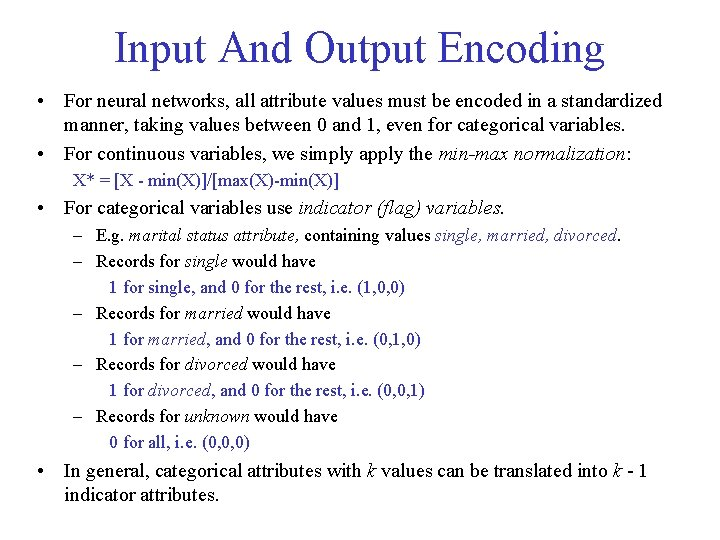Input And Output Encoding • For neural networks, all attribute values must be encoded in a standardized manner, taking values between 0 and 1, even for categorical variables. • For continuous variables, we simply apply the min-max normalization: X* = [X - min(X)]/[max(X)-min(X)] • For categorical variables use indicator (flag) variables. – E. g. marital status attribute, containing values single, married, divorced. – Records for single would have 1 for single, and 0 for the rest, i. e. (1, 0, 0) – Records for married would have 1 for married, and 0 for the rest, i. e. (0, 1, 0) – Records for divorced would have 1 for divorced, and 0 for the rest, i. e. (0, 0, 1) – Records for unknown would have 0 for all, i. e. (0, 0, 0) • In general, categorical attributes with k values can be translated into k - 1 indicator attributes.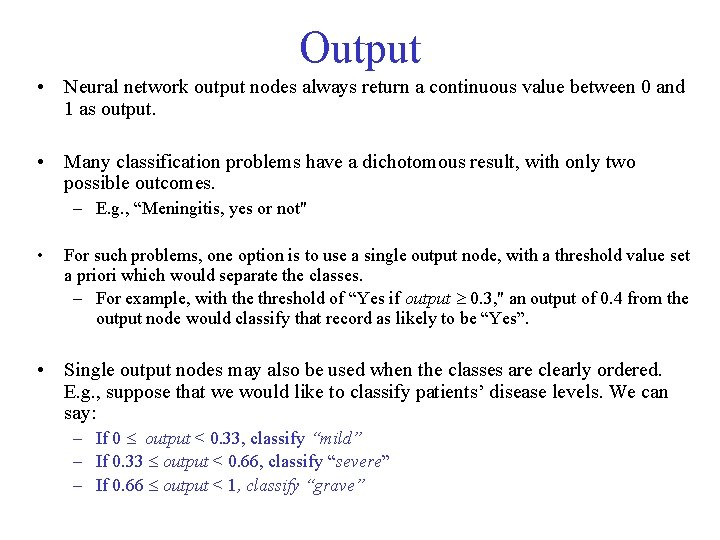Output • Neural network output nodes always return a continuous value between 0 and 1 as output. • Many classification problems have a dichotomous result, with only two possible outcomes. – E. g. , “Meningitis, yes or not" • For such problems, one option is to use a single output node, with a threshold value set a priori which would separate the classes. – For example, with the threshold of “Yes if output 0. 3, " an output of 0. 4 from the output node would classify that record as likely to be “Yes”. • Single output nodes may also be used when the classes are clearly ordered. E. g. , suppose that we would like to classify patients’ disease levels. We can say: – If 0 output < 0. 33, classify “mild” – If 0. 33 output < 0. 66, classify “severe” – If 0. 66 output < 1, classify “grave”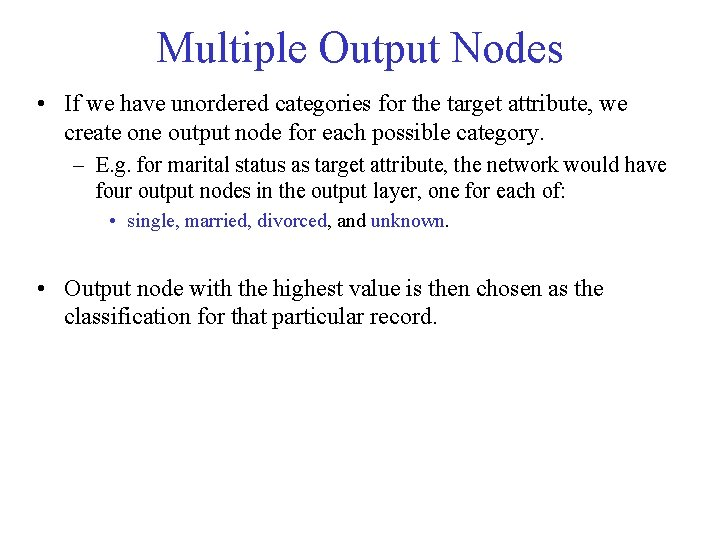Multiple Output Nodes • If we have unordered categories for the target attribute, we create one output node for each possible category. – E. g. for marital status as target attribute, the network would have four output nodes in the output layer, one for each of: • single, married, divorced, and unknown. • Output node with the highest value is then chosen as the classification for that particular record.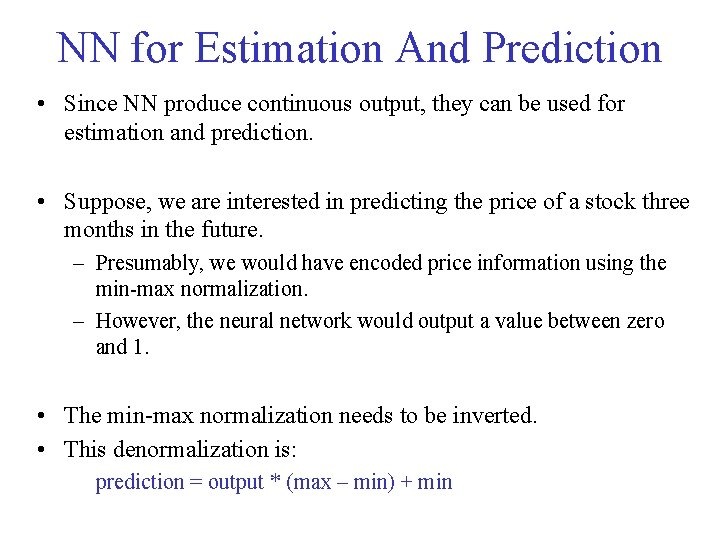NN for Estimation And Prediction • Since NN produce continuous output, they can be used for estimation and prediction. • Suppose, we are interested in predicting the price of a stock three months in the future. – Presumably, we would have encoded price information using the min-max normalization. – However, the neural network would output a value between zero and 1. • The min-max normalization needs to be inverted. • This denormalization is: prediction = output * (max – min) + min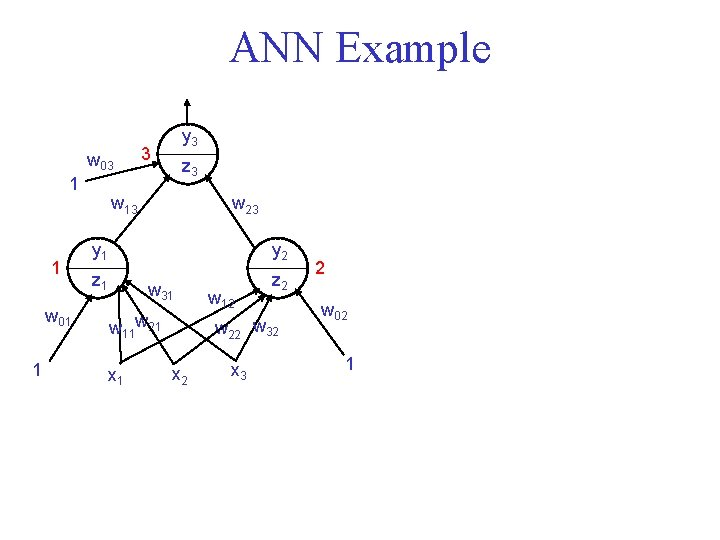ANN Example w 03 1 1 w 01 1 y 3 3 z 3 w 13 w 23 y 1 y 2 z 1 z 2 w 31 w 11 w 21 x 1 w 12 w 22 w 32 x 3 2 w 02 1Learning Weights For an output unit p we similarly have: w 03 1 1 w 01 1 y 3 3 p=3 in this example z 3 w 13 w 23 y 1 y 2 z 1 z 2 w 31 w 11 w 21 x 1 w 12 w 22 w 32 x 3 2 w 02 1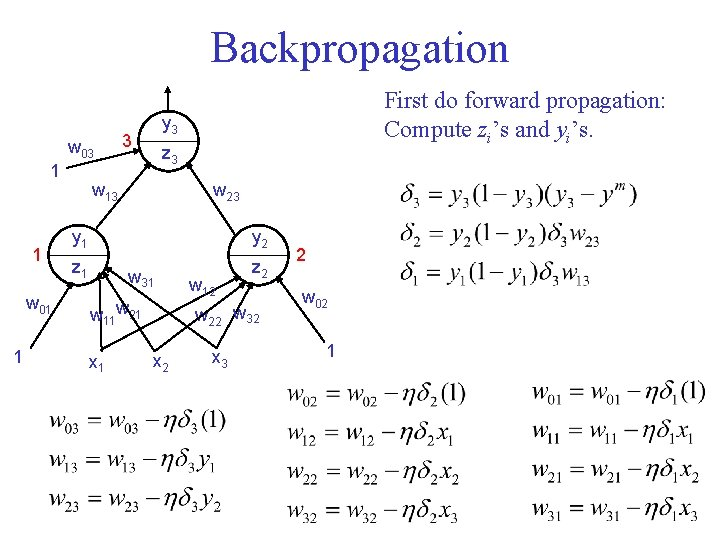Backpropagation w 03 1 1 w 01 1 First do forward propagation: Compute zi’s and yi’s. y 3 3 z 3 w 13 w 23 y 1 y 2 z 1 z 2 w 31 w 11 w 21 x 1 w 12 w 22 w 32 x 3 2 w 02 1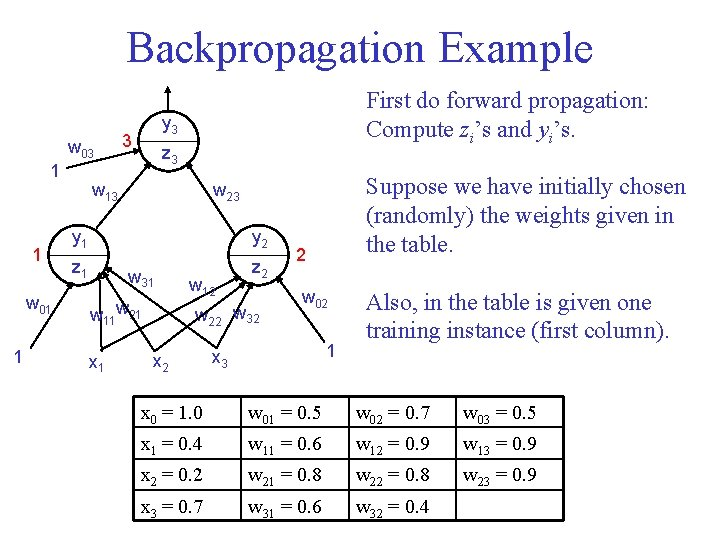Backpropagation Example w 03 1 1 w 01 1 First do forward propagation: Compute zi’s and yi’s. y 3 3 z 3 w 13 y 1 y 2 z 1 z 2 w 31 w 11 w 21 x 1 Suppose we have initially chosen (randomly) the weights given in the table. w 23 w 12 w 22 w 32 x 2 2 w 02 1 x 3 Also, in the table is given one training instance (first column). x 0 = 1. 0 w 01 = 0. 5 w 02 = 0. 7 w 03 = 0. 5 x 1 = 0. 4 w 11 = 0. 6 w 12 = 0. 9 w 13 = 0. 9 x 2 = 0. 2 w 21 = 0. 8 w 22 = 0. 8 w 23 = 0. 9 x 3 = 0. 7 w 31 = 0. 6 w 32 = 0. 4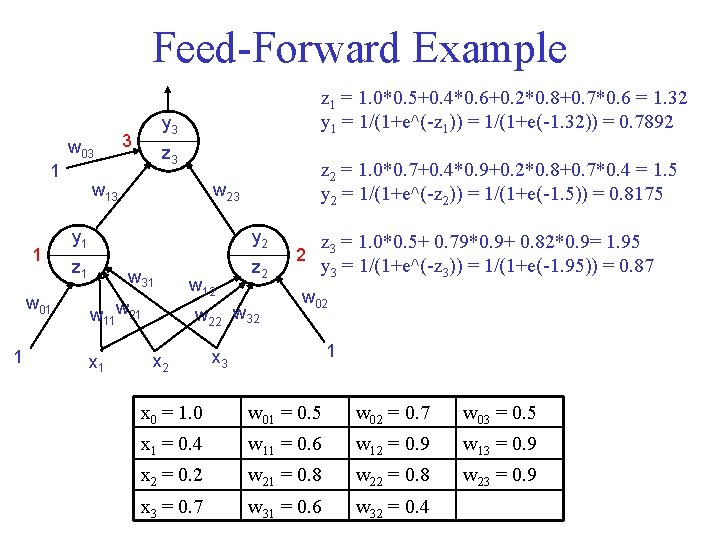Feed-Forward Example w 03 1 1 w 01 1 z 1 = 1. 0*0. 5+0. 4*0. 6+0. 2*0. 8+0. 7*0. 6 = 1. 32 y 1 = 1/(1+e^(-z 1)) = 1/(1+e(-1. 32)) = 0. 7892 y 3 3 z 3 w 13 z 2 = 1. 0*0. 7+0. 4*0. 9+0. 2*0. 8+0. 7*0. 4 = 1. 5 y 2 = 1/(1+e^(-z 2)) = 1/(1+e(-1. 5)) = 0. 8175 w 23 y 1 y 2 z 1 w 31 w 11 w 21 x 1 w 12 z 2 w 22 w 32 x 2 2 z 3 = 1. 0*0. 5+ 0. 79*0. 9+ 0. 82*0. 9= 1. 95 y 3 = 1/(1+e^(-z 3)) = 1/(1+e(-1. 95)) = 0. 87 w 02 1 x 3 x 0 = 1. 0 w 01 = 0. 5 w 02 = 0. 7 w 03 = 0. 5 x 1 = 0. 4 w 11 = 0. 6 w 12 = 0. 9 w 13 = 0. 9 x 2 = 0. 2 w 21 = 0. 8 w 22 = 0. 8 w 23 = 0. 9 x 3 = 0. 7 w 31 = 0. 6 w 32 = 0. 4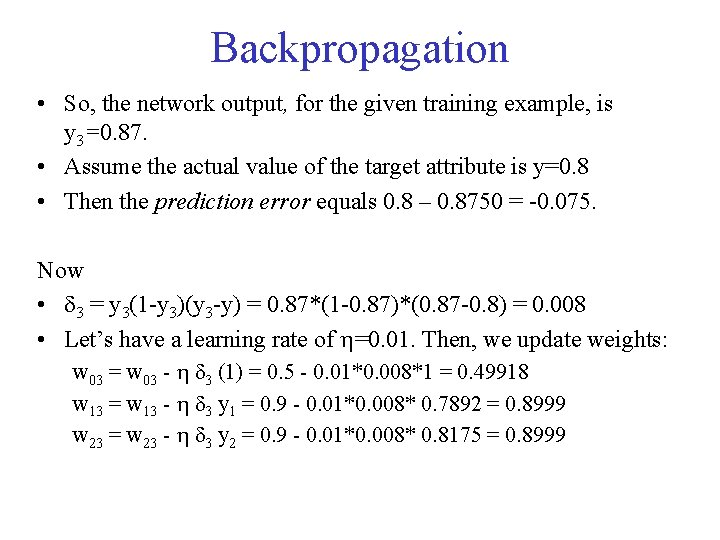Backpropagation • So, the network output, for the given training example, is y 3=0. 87. • Assume the actual value of the target attribute is y=0. 8 • Then the prediction error equals 0. 8 – 0. 8750 = -0. 075. Now • 3 = y 3(1 -y 3)(y 3 -y) = 0. 87*(1 -0. 87)*(0. 87 -0. 8) = 0. 008 • Let’s have a learning rate of =0. 01. Then, we update weights: w 03 = w 03 - 3 (1) = 0. 5 - 0. 01*0. 008*1 = 0. 49918 w 13 = w 13 - 3 y 1 = 0. 9 - 0. 01*0. 008* 0. 7892 = 0. 8999 w 23 = w 23 - 3 y 2 = 0. 9 - 0. 01*0. 008* 0. 8175 = 0. 8999Backpropagation • 2 = y 2(1 -y 2) 3 w 23 = 0. 8175*(1 -0. 8175)*0. 008*0. 9 = 0. 001 • 1 = y 1(1 -y 1) 3 w 13 = 0. 7892*(1 - 0. 7892)*0. 008*0. 9 = 0. 0012 • Then, we update weights: w 02 = w 02 - 2 (1) = 0. 7 - 0. 01*0. 001*1 = 0. 6999 w 12 = w 12 - 2 x 1 = 0. 9 - 0. 01*0. 001* 0. 4 = 0. 8999 w 22 = w 22 - 2 x 2 = 0. 8 - 0. 01*0. 001* 0. 2 = 0. 7999 w 32 = w 32 - 2 x 3 = 0. 4 - 0. 01*0. 001* 0. 7 = 0. 3999 w 01 = w 01 - 1 (1) = 0. 5 - 0. 01*0. 001*1 = 0. 4999 w 11 = w 11 - 1 x 1 = 0. 6 - 0. 01*0. 001* 0. 4 = 0. 5999 w 21 = w 21 - 1 x 2 = 0. 8 - 0. 01*0. 001* 0. 2 = 0. 7999 w 31 = w 31 - 1 x 3 = 0. 6 - 0. 01*0. 001* 0. 7 = 0. 5999